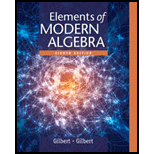# (See Exercise 38.) Suppose that n &gt; 1 and that d = ( a , n ) is a divisor of b . Let a = a 0 d , n = n 0 d , and b = b 0 d , where a 0 , n 0 , and b 0 are integers. The following statements a − e lead to a proof that the congruence a x ≡ b ( m o d n ) has exactly d incongruent solutions modulo n , and they show how such a set of solutions can be found. a. Prove that a x ≡ b ( m o d n ) if and only if a 0 x ≡ b 0 ( m o d n 0 ) . b. Prove that if x 1 and x 2 are any two solutions to a 0 x ≡ b 0 ( m o d n 0 ) , then it follows that x 1 ≡ x 2 ( m o d n 0 ) . c. Let x 1 be a fixed solution to a 0 x ≡ b 0 ( m o d n 0 ) , and prove that each of the d inte-gers in the list x 1 , x 1 + n 0 , x 1 + 2 n 0 , . . . , x 1 + ( d − 1 ) n 0 is a solution to a x ≡ b ( m o d n ) . d. Prove that no two of the solutions listed in part c are congruent modulo n . e. Prove that any solution to a x ≡ b ( m o d n ) is congruent to one of the numbers listed in part c . Let d = ( a , n ) where n &gt; 1 . Prove that if there is a solution to a x ≡ b ( m o d n ) , then d divides b .### Elements Of Modern Algebra

8th Edition
Gilbert + 2 others
Publisher: Cengage Learning,
ISBN: 9781285463230### Elements Of Modern Algebra

8th Edition
Gilbert + 2 others
Publisher: Cengage Learning,
ISBN: 9781285463230

#### Solutions

Chapter
Section
Chapter 2.5, Problem 39E
Textbook Problem

## (See Exercise 38.) Suppose that n > 1 and that d = ( a ,   n ) is a divisor of b . Let a = a 0 d , n = n 0 d , and b = b 0 d , where a 0 , n 0 , and b 0 are integers. The following statements a − e lead to a proof that the congruence a x ≡ b ( m o d   n ) has exactly d incongruent solutions modulo n , and they show how such a set of solutions can be found.a. Prove that a x ≡ b ( m o d   n ) if and only if a 0 x ≡ b 0 ( m o d   n 0 ) .b. Prove that if x 1 and x 2 are any two solutions to a 0 x ≡ b 0 ( m o d   n 0 ) , then it follows that x 1 ≡ x 2 ( m o d   n 0 ) .c. Let x 1 be a fixed solution to a 0 x ≡ b 0 ( m o d   n 0 ) , and prove that each of the d inte-gers in the list x 1 , x 1 + n 0 , x 1 + 2 n 0 ,   .   .   .   , x 1 + ( d − 1 ) n 0 is a solution to a x ≡ b ( m o d   n ) .d. Prove that no two of the solutions listed in part   c are congruent modulo n .e. Prove that any solution to a x ≡ b ( m o d   n ) is congruent to one of the numbers listed in part c .Let d   =   ( a ,   n ) where n > 1 . Prove that if there is a solution to a x ≡ b ( m o d   n ) , then d divides b .

Expert Solution

(a)

To determine

To prove: axb(modn), if and only if a0xb0(modn0).

### Explanation of Solution

Given information:

n>1 and that d=(a,n) is a divisor of b.

a=a0d,n=n0d, and b=b0d where a0,n0 and b0 are integers.

Formula used:

1) Definition: Congruence Modulo n

Let n be a positive integer, n>1. For integers x and y, x is congruent to y modulo n, if and only if xy is a multiple of n. We write xy(modn) to indicate that x is congruent to y

modulo n.

2) Theorem: The Division Algorithm:

Let a and b be integers with b>0. Then, there exist unique integers q and r, such that

a=bq+r with 0r<b.

Proof:

Suppose that n>1 and that d=(a,n) is a divisor of b.

Let a=a0d,n=n0d, and b=b0d, where a0,n0 and b0 are integers.

Let axb(modn)

Expert Solution

(b)

To determine

To prove: If x1 and x2 are any two solutions to a0xb0(modn0), then it follows that x1x2(modn0).

Expert Solution

(c)

To determine

To prove: If x1 is a fixed solution to a0xb0(modn0), then each of the integer in the list

x1,x1+n0,x1+2n0,,x1+(d1)n0 is a solution to axb(modn).

Expert Solution

(d)

To determine

To prove: No two solutions in the list x1,x1+n0,x1+2n0,,x1+(d1)n0 are congruent modulo n.

Expert Solution

(e)

To determine

To prove: Any solution to axb(modn) is congruent to one of the numbers in the list x1,x1+n0,x1+2n0,,x1+(d1)n0.

### Want to see the full answer?

Check out a sample textbook solution.See solution

### Want to see this answer and more?

Bartleby provides explanations to thousands of textbook problems written by our experts, many with advanced degrees!

See solution